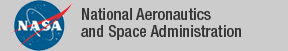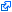### Top File of PVS graphs Library

```
%------------------------------------------------------------------------------
%
% Graph Theory
%
%  Authors:
%
%      Jon Sjogren       AFOSR
%      Ricky W. Butler   NASA Langley
%
%  Version 2.1           UPDATED to PVS 2.2: 11/17/98
%  Version 2.2           cycle_deg, tree_paths extended  12/20/04
%  Version 2.3           matroids and mappings added     7/19/2005
%  Version 2.4           Jon Sjogren has generalized Menger's theorem
%                        for k independent paths (not just 2)           7/12/2007
%                        See k_menger.pvs and menger.pvs
%
%
%  Maintained by:
%
%     Rick Butler        NASA Langley Research Center
%                        R.W.Butler@larc.nasa.gov
%
%  abstract_min          -- abstract definition of min
%  abstract_max          -- abstract definition of max
%  circuit_deg           -- degree of circuits
%  circuits              -- theory of circuits
%  cycle_deg             -- theorem about degree and existence of cycle
%  doubletons            -- theory of doubletons used for definition of edge
%  graphs                -- fundamental definition of a graph
%  graph_complected      -- unusual definition of connected graph
%  graph_conn_defs       -- defs of piece, path, and structural connectedness
%  graph_conn_piece      -- structural connected ==> piece connected
%  graph_connected       -- all connected defs are equivalent
%  graph_path_conn       -- path connected ==> structural connected
%  graph_piece_path      -- piece connected ==> path connected
%  graph_deg             -- definition of degree
%  graph_deg_sum         -- theorem relating vertex degree and number of edges
%  graph_inductions      -- vertex and edge inductions for graphs
%  graph_ops             -- delete vertex and delete edge operations
%  ind_paths             -- definition of independent paths
%  max_subgraphs         -- maximal subgraphs with specified property
%  max_subtrees          -- maximal subtrees with specified property
%  min_walk_reduced      -- theorem that minimum walk is reduced
%  min_walks             -- minimum walk satisfying a property
%  path_lems             -- some useful lemmas about paths
%  path_ops              -- deleting vertex and edge operations
%  paths                 -- fundamental definition and properties about paths
%  ramsey_new            -- Ramsey's theorem
%  reduce_walks          -- operation to reduce a walk
%  sep_set_lems          -- properties of separating sets
%  sep_sets              -- definition of separating sets
%  subgraphs             -- generation of subgraphs from vertex sets
%  subgraphs_from_walk   -- generation of subgraphs from walks
%  subtrees              -- subtrees of a graph
%  tree_circ             -- theorem that tree has no circuits
%  tree_paths            -- theorem that tree has only one path between vertices
%  trees                 -- fundamental definition of trees
%  walk_inductions       -- induction on length of a walk
%  walks                 -- fundamental definition and properties of walks
%  NEW
%    k_menger,
%    complem,
%    graph_pair,
%    mappings
%    menger              -- menger's theorem now fully general (by Jon Sjogren 2007)
%
%  The FOLLOWING theories are now obsolete:
%
%    old_menger
%    h_menger              -- hard menger
%    meng_scaff            -- scaffolding for hard menger proof
%    meng_scaff_defs       -- scaffolding for hard menger proof
%    meng_scaff_prelude    -- scaffolding for hard menger proof
%-------------------------------------------------------------------------------
top: THEORY

BEGIN

IMPORTING graphs,
graph_deg,
graph_deg_sum,
walks,
paths,
path_ops,
path_lems,
path_circ,
circuits,
subgraphs,
subgraphs_from_walk,
graph_ops,
graph_from_edges,
max_subgraphs,
max_subtrees,
trees,
tree_circ,
subtrees,
graph_connected,
graph_inductions,
walk_inductions,
ramsey_new,
min_walk_reduced,
reduce_walks,
menger,
tree_paths,
circuit_deg,
cycle_deg,

abstract_min      ,
abstract_max      ,
doubletons        ,
graph_complected  ,
graph_conn_defs   ,
graph_conn_piece  ,
graph_connected   ,
graph_path_conn   ,
graph_piece_path  ,
graph_inductions  ,
ind_paths         ,
old_menger        ,  %% obsolete
h_menger          ,  %% obsolete
meng_scaff        ,  %% obsolete
meng_scaff_defs   ,  %% obsolete
meng_scaff_prelude,  %% obsolete
menger            ,
min_walks         ,
sep_set_lems      ,
sep_sets          ,
complem,
k_menger,
mantel,              %% Mantel's Theorem (A. Dutle)
graph_pair,
mappings,
matroids             %% experimental

END top

```
The tagidentifies links that are outside the NASA domain